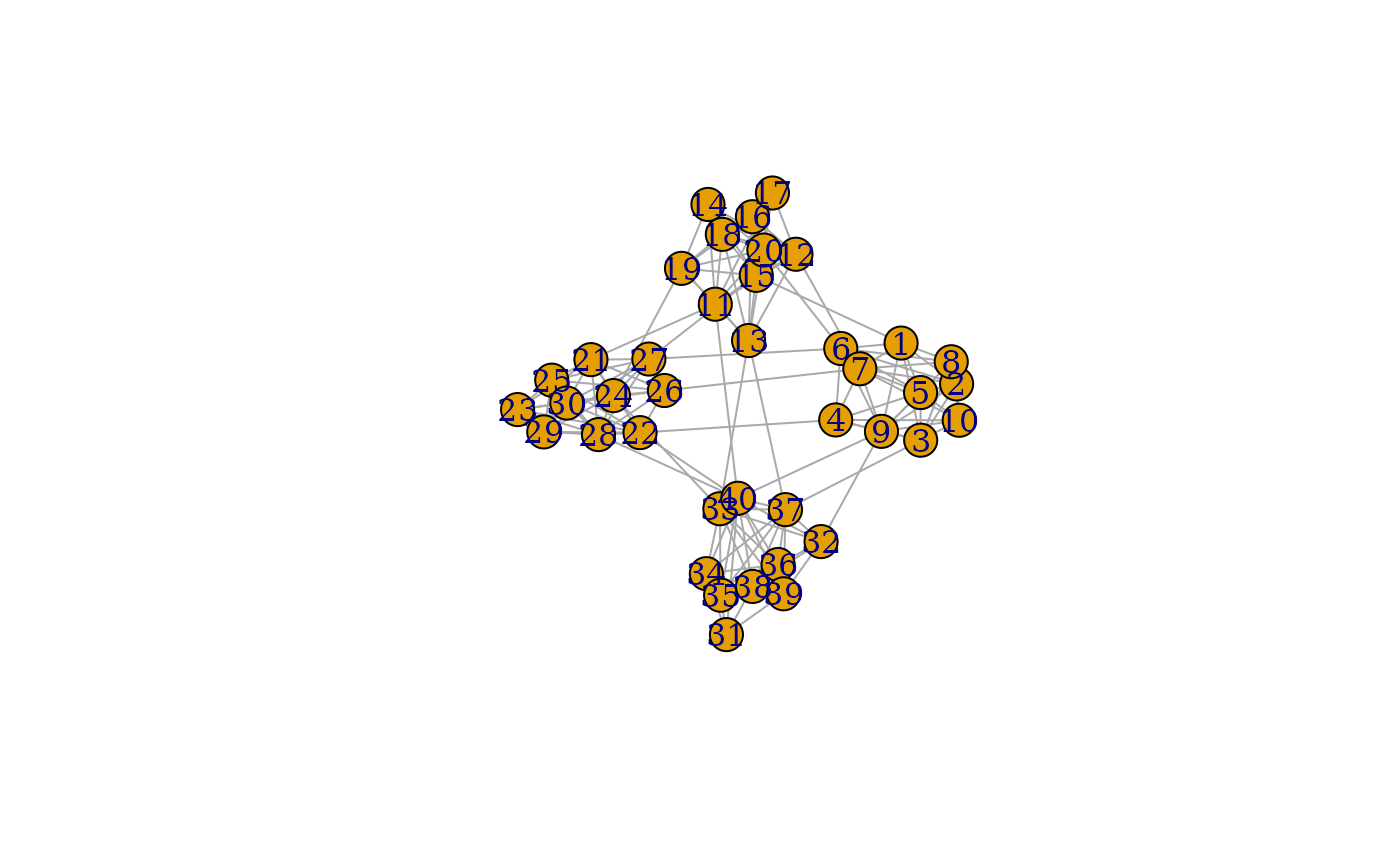This set of graph creation algorithms simulate the topology by, in some way, connecting subgraphs. The nature of their algorithm is described in detail at the linked igraph documentation.

play_blocks(n, size_blocks, p_between, directed = TRUE, loops = FALSE)

play_blocks_hierarchy(n, size_blocks, rho, p_within, p_between)

play_islands(n_islands, size_islands, p_within, m_between)

play_smallworld(
n_dim,
dim_size,
order,
p_rewire,
loops = FALSE,
multiple = FALSE
)

## Arguments

n The number of nodes in the graph. The number of vertices in each block The probability of edges within and between groups/blocks Should the resulting graph be directed Are loop edges allowed The fraction of vertices per cluster The number of densely connected islands The number of nodes in each island The number of edges between groups/islands The dimension and size of the starting lattice The neighborhood size to create connections from The rewiring probability of edges Are multiple edges allowed

## Value

A tbl_graph object

## Functions

• play_blocks: Create graphs by sampling from stochastic block model. See igraph::sample_sbm()

• play_blocks_hierarchy: Create graphs by sampling from the hierarchical stochastic block model. See igraph::sample_hierarchical_sbm()

• play_islands: Create graphs with fixed size and edge probability of subgraphs as well as fixed edge count between subgraphs. See igraph::sample_islands()

• play_smallworld: Create graphs based on the Watts-Strogatz small- world model. See igraph::sample_smallworld()

Other graph games: evolution_games, sampling_games, type_games
plot(play_islands(4, 10, 0.7, 3))